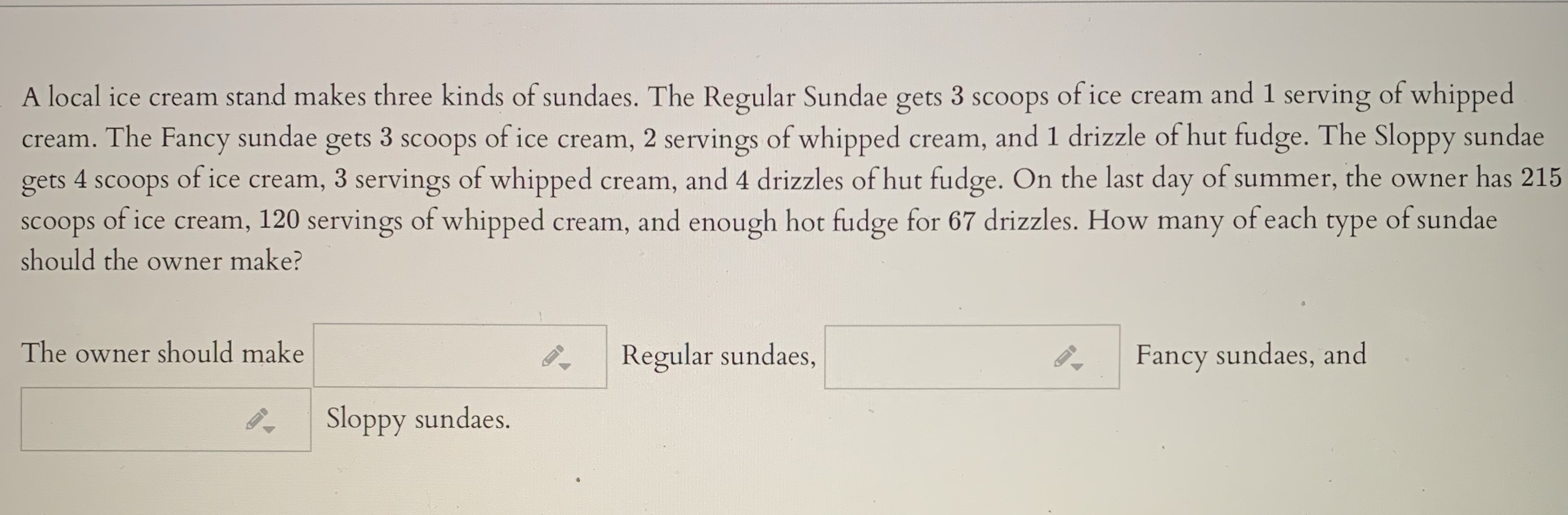### Still have math questions?

Algebra
QuestionA local ice cream stand makes three kinds of sundaes. The Regular Sundae gets $$3$$ scoops of ice cream and $$1$$ serving of whipped cream. The Fancy sundae gets $$3$$ scoops of ice cream, $$2$$ servings of whipped cream, and $$1$$ drizzle of hut fudge. The Sloppy sundae gets $$4$$ scoops of ice cream, $$3$$ servings of whipped cream, and $$4$$ drizzles of hut fudge. On the last day of summer, the owner has $$215$$ scoops of ice cream, $$120$$ servings of whipped cream, and enough hot fudge for $$67$$ drizzles. How many of each type of sundae should the owner make?

The owner should make $$\square$$ Regular sundaes, $$\square$$ Fancy sundaes, and $$\square$$ Sloppy sundaes.